# Permeability (electromagnetism) facts for kids

Kids Encyclopedia Facts
Magnetic Circuits

Conventional Magnetic Circuits
• Magnetomotive force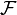$\mathcal F$
• Magnetic flux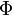$\Phi$
• Magnetic reluctance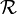$\mathcal R$

Phasor Magnetic Circuits
• Complex reluctance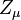$Z_\mu$

Related Concepts
• Magnetic permeability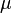$\mu$

Gyrator-capacitor model variables
• Magnetic impedance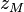$z_M$
• Effective resistance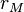$r_M$
• Magnetic inductivity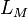$L_M$
• Magnetic capacitivity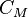$C_M$

Permeability is a property of a material that describes how dense a magnetic field would be if the same amount of current was passed through it. Permeability is measured in henries per metre (H/m) and its symbol is$\mu$.

Since empty space has a constant permeability (called the permeability of free space or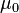$\mu_{0}$) of exactly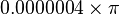$0.0000004 \times \pi$, most materials are listed with a relative permeability (symbol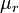$\mu_{r}$). Relative permeability is the permeability of the material divided by the permeability of free space (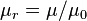$\mu_{r} = \mu / \mu_{0}$). The permeability of most materials is very close to 1. That means that the permeability of most materials is close enough that we can typically ignore it and use the permeability of free space instead. The biggest exceptions are materials called ferromagnetic materials. Some examples are iron (5000) and nickel (600). Some materials have been specially designed to have a permeability one million times larger than empty space.Permeability (electromagnetism) Facts for Kids. Kiddle Encyclopedia.